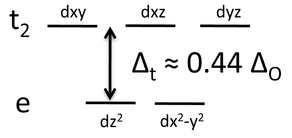# 4.12: Tetrahedral Complexes

$$\newcommand{\vecs}{\overset { \rightharpoonup} {\mathbf{#1}} }$$ $$\newcommand{\vecd}{\overset{-\!-\!\rightharpoonup}{\vphantom{a}\smash {#1}}}$$$$\newcommand{\id}{\mathrm{id}}$$ $$\newcommand{\Span}{\mathrm{span}}$$ $$\newcommand{\kernel}{\mathrm{null}\,}$$ $$\newcommand{\range}{\mathrm{range}\,}$$ $$\newcommand{\RealPart}{\mathrm{Re}}$$ $$\newcommand{\ImaginaryPart}{\mathrm{Im}}$$ $$\newcommand{\Argument}{\mathrm{Arg}}$$ $$\newcommand{\norm}{\| #1 \|}$$ $$\newcommand{\inner}{\langle #1, #2 \rangle}$$ $$\newcommand{\Span}{\mathrm{span}}$$ $$\newcommand{\id}{\mathrm{id}}$$ $$\newcommand{\Span}{\mathrm{span}}$$ $$\newcommand{\kernel}{\mathrm{null}\,}$$ $$\newcommand{\range}{\mathrm{range}\,}$$ $$\newcommand{\RealPart}{\mathrm{Re}}$$ $$\newcommand{\ImaginaryPart}{\mathrm{Im}}$$ $$\newcommand{\Argument}{\mathrm{Arg}}$$ $$\newcommand{\norm}{\| #1 \|}$$ $$\newcommand{\inner}{\langle #1, #2 \rangle}$$ $$\newcommand{\Span}{\mathrm{span}}$$$$\newcommand{\AA}{\unicode[.8,0]{x212B}}$$

Tetrahedral complexes are formed with late transition metal ions (Co2+, Cu2+, Zn2+, Cd2+) and some early transition metals (Ti4+, Mn2+), especially in situations where the ligands are large. In these cases the small metal ion cannot easily accommodate a coordination number higher than four. Examples of tetrahedal ions and molecules are [CoCl4]2-, [MnCl4]2-, and TiX4 (X = halogen). Tetrahedral coordination is also observed in some oxo-anions such as [FeO4]4-, which exists as discrete anions in the salts Na4FeO4 and Sr2FeO4, and in the neutral oxides RuO4 and OsO4. The metal carbonyl complexes Ni(CO)4 and Co(CO)4]- are also tetrahedral.The splitting of the d-orbitals in a tetrahedral crystal field can be understood by connecting the vertices of a tetrahedron to form a cube, as shown in the picture at the left. The tetrahedral M-L bonds lie along the body diagonals of the cube. The dz2 and dx2-y2 orbitals point along the cartesian axes, i.e., towards the faces of the cube, and have the least contact with the ligand lone pairs. Therefore these two orbitals form a low energy, doubly degenerate e set. The dxy, dyz, and dxz orbitals point at the edges of the cube and form a triply degenerate t2 set. While the t2 orbitals have more overlap with the ligand orbitals than the e set, they are still weakly interacting compared to the eg orbitals of an octahedral complex.

The resulting crystal field energy diagram is shown at the right. The splitting energy, Δt, is about 4/9 the splitting of an octahedral complex formed with the same ligands. For 3d elements, Δt is thus small compared to the pairing energy and their tetrahedral complexes are always high spin. Note that we have dropped the "g" subscript because the tetrahedron does not have a center of symmetry.Tetrahedral complexes often have vibrant colors because they lack the center of symmetry that forbids a d-d* transition. Because the low energy transition is allowed, these complexes typically absorb in the visible range and have extinction coefficients that are 1-2 orders of magnitude higher than the those of the corresponding octahedral complexes. An illustration of this effect can be seen in Drierite, which contains particles of colorless, anhydrous calcium sulfate (gypsum) that absorbs moisture from gases. The indicator dye in Drierite is cobalt (II) chloride, which is is a light pink when wet (octahedral) and deep blue when dry (tetrahedral). The reversible hydration reaction is:

$\ce{Co[CoCl4] + 12H2O -> 2 Co(H2O)6Cl2}$

(deep blue, tetrahedral CoCl42-) (light pink, octahedral [Co(H2O)6]2+)4.12: Tetrahedral Complexes is shared under a CC BY-SA license and was authored, remixed, and/or curated by LibreTexts.